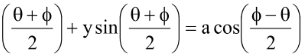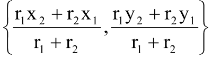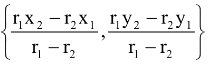# Pair of Tangents

## Circles of Class 11

### Pair of Tangents

Tangents are drawn from P(x1, y1) to the circle S = x2 + y2 + 2gx + 2fy + c = 0.

The joint equation of the pair of tangents is SS1 = T2 where

S = x2 + y2 + 2gx + 2fy + c

S1 = x12 + y12 + 2gx1 + 2fy1 + c

and T = xx1 + yy1 + g(x + x1) + f(y + y1) + c

Equation of the chord joining two points (acosθ, a sinθ) and (acosφ, asinφ) on the circle x2 + y2 = a2 is

x cosNote that In case θ → φ it becomes a tangent given by

xcosφ + y sinφ = a

## External and Internal contacts of circles

Two circles with centers C1(x1, y1) and C2 (x2, y2) and radii r1, r2 respectively touch each other.

(a) Externally

If |C1C2| = r1 + r2 and the point of contact is(b) Internally

If |C1C2| = |r1 – r2| and the point of contact is